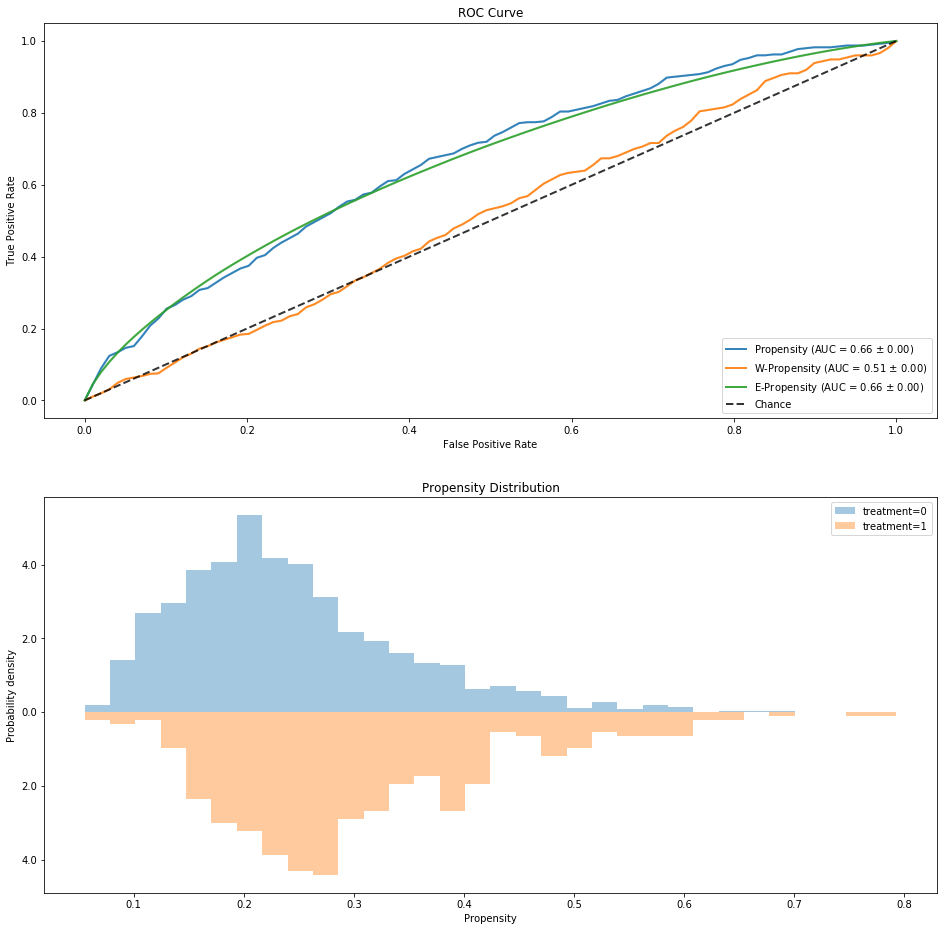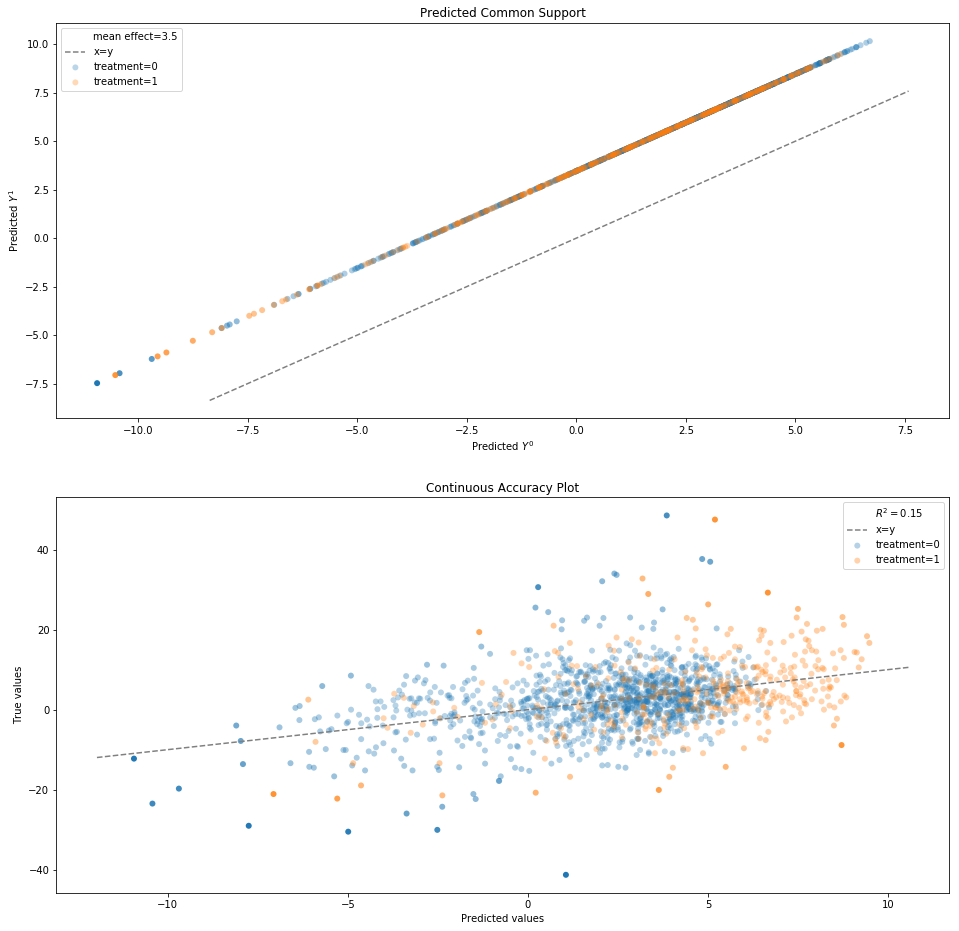# Doubly Robust Models¶

Basically, different ensemble models that utilize a weight model to augment the outcome model.

This notebook presents different combinations of mixing outcome and propensity models,
but since the possible combination are a lot, it does not intend to show all of them.

In :
%matplotlib inline
from sklearn.linear_model import LogisticRegression, LinearRegression
from causallib.estimation import IPW, Standardization, StratifiedStandardization
from causallib.estimation import AIPW, PropensityFeatureStandardization, WeightedStandardization
from causallib.evaluation import PropensityEvaluator, OutcomeEvaluator


#### Data:¶

The effect of quitting to smoke on weight loss.
Data example is taken from Hernan and Robins Causal Inference Book

In :
data = load_nhefs()

Out:
age race sex smokeintensity smokeyrs wt71 active_1 active_2 education_2 education_3 education_4 education_5 exercise_1 exercise_2 age^2 wt71^2 smokeintensity^2 smokeyrs^2 qsmk wt82_71
0 42 1 0 30 29 79.04 0 0 0 0 0 0 0 1 1764 6247.3216 900 841 0 -10.093960
1 36 0 0 20 24 58.63 0 0 1 0 0 0 0 0 1296 3437.4769 400 576 0 2.604970
2 56 1 1 20 26 56.81 0 0 1 0 0 0 0 1 3136 3227.3761 400 676 0 9.414486
3 68 1 0 3 53 59.42 1 0 0 0 0 0 0 1 4624 3530.7364 9 2809 0 4.990117
4 40 0 0 20 19 87.09 1 0 1 0 0 0 1 0 1600 7584.6681 400 361 0 4.989251

## Vanilla Doubly Robust¶

Used for average outcomes.
Its individual outcome estimation is directly its outcome model one's,
but for population outcome, it corrects the observed outcome using the individual outcome prediction before taking weighted average.

In :
ipw = IPW(LogisticRegression(solver="liblinear"), clip_min=0.05, clip_max=0.95)
std = StratifiedStandardization(LinearRegression())
dr = AIPW(std, ipw)
dr.fit(data.X, data.a, data.y)

Out:
AIPW(outcome_covariates=None, outcome_model=StratifiedStandardization(learner=LinearRegression(copy_X=True, fit_intercept=True, n_jobs=None,
normalize=False)), predict_proba=False, weight_covariates=None,
weight_model=IPW(clip_min=0.05, clip_max=0.95, use_stabilized=False,
learner=LogisticRegression(C=1.0, class_weight=None, dual=False, fit_intercept=True,
intercept_scaling=1, max_iter=100, multi_class='warn',
n_jobs=None, penalty='l2', random_state=None, solver='liblinear',
tol=0.0001, verbose=0, warm_start=False)))

Doubly-robust corrected population outcomes:

In :
pop_outcome = dr.estimate_population_outcome(data.X, data.a, data.y)
pop_outcome

Fraction of values being truncated: 0.00000.

Out:
qsmk
0    1.772502
1    5.185455
dtype: float64
In :
effect = dr.estimate_effect(pop_outcome, pop_outcome)
effect

Out:
diff    3.412954
dtype: float64

## Doubly Robust IP-Feature¶

Trains a weight model, and then use its output (predicted weights) as additional features to the outcome model.

If possible (like in IPW) the entire weight-matrix (weight of each individual for each treatment value) is used,
but usually, only a weight vector (according to the actual treatment assignment) is used.

In :
ipw = IPW(LogisticRegression(solver="liblinear"))
std = Standardization(LinearRegression())
dr = PropensityFeatureStandardization(std, ipw)
dr.fit(data.X, data.a, data.y)

Out:
PropensityFeatureStandardization(outcome_covariates=None, outcome_model=Standardization(encode_treatment=False, predict_proba=False,
learner=LinearRegression(copy_X=True, fit_intercept=True, n_jobs=None,
normalize=False)), predict_proba=False, weight_covariates=None,
weight_model=IPW(clip_min=None, clip_max=None, use_stabilized=False,
learner=LogisticRegression(C=1.0, class_weight=None, dual=False, fit_intercept=True,
intercept_scaling=1, max_iter=100, multi_class='warn',
n_jobs=None, penalty='l2', random_state=None, solver='liblinear',
tol=0.0001, verbose=0, warm_start=False)))
In :
ind_outcomes = dr.estimate_individual_outcome(data.X, data.a)

Out:
qsmk 0 1
0 4.313683 7.773268
1 6.380521 9.840105
2 2.020079 5.479664
3 -4.285034 -0.825450
4 2.278624 5.738208
In :
effect = dr.estimate_effect(ind_outcomes, ind_outcomes,
effect_types=["diff", "ratio"])
effect

Out:
diff     3.459584
ratio    2.979170
dtype: float64

## Doubly Robust Joffe¶

This uses an importance sampling using the estimated weights.

On the first step weight model is trained and used to predict weights.
These predicted weights are then provided as sample_weights to the outcome model.

In :
ipw = IPW(LogisticRegression(solver="liblinear"))
std = Standardization(LinearRegression())
dr = WeightedStandardization(std, ipw)
dr.fit(data.X, data.a, data.y)

Out:
WeightedStandardization(outcome_covariates=None, outcome_model=Standardization(encode_treatment=False, predict_proba=False,
learner=LinearRegression(copy_X=True, fit_intercept=True, n_jobs=None,
normalize=False)), predict_proba=False, weight_covariates=None,
weight_model=IPW(clip_min=None, clip_max=None, use_stabilized=False,
learner=LogisticRegression(C=1.0, class_weight=None, dual=False, fit_intercept=True,
intercept_scaling=1, max_iter=100, multi_class='warn',
n_jobs=None, penalty='l2', random_state=None, solver='liblinear',
tol=0.0001, verbose=0, warm_start=False)))
In :
ind_outcomes = dr.estimate_individual_outcome(data.X, data.a)

Out:
qsmk 0 1
0 4.118489 7.588472
1 7.010648 10.480631
2 3.403031 6.873014
3 -4.624051 -1.154068
4 3.125411 6.595394
In :
pop_outcome = dr.estimate_population_outcome(data.X, data.a)
pop_outcome

Out:
qsmk
0    1.777886
1    5.247869
dtype: float64
In :
effect = dr.estimate_effect(pop_outcome, pop_outcome)
effect

Out:
diff    3.469983
dtype: float64

## Confounders, Instruments and Effect Modifiers¶

On general there are three main types of covariates in a graphical causal model:

1. Confounders: variables that affect both the outcome and treatment
2. Instruments: variables that affect the treatment assignment but not the outcome.
3. Effect mods: variables that affect the outcome but not the treatment assignment

For a Doubly Robust model that holds both outcome model and weight (treatment assignment prediction) model,
These can specified by a list of covariates outcome_covariates and weight_covariates,
which their intersection correspond to confounders and their symmetric difference are the effect modifiers and instruments, respectively.

In :
# Say smoke quitting does not depend on your weight and on your age
weight_covariates = [col for col in data.X.columns
if not col.startswith("wt") and not col.startswith("age")]
ipw = IPW(LogisticRegression(solver="liblinear"))
std = Standardization(LinearRegression())
dr = PropensityFeatureStandardization(std, ipw,
weight_covariates=weight_covariates)
# By not specifying outcome_covariates the model will use all covariates
dr.fit(data.X, data.a, data.y);

In :
pop_outcome = dr.estimate_population_outcome(data.X, data.a)
pop_outcome

Out:
qsmk
0    1.750101
1    5.201514
dtype: float64
In :
dr.estimate_effect(pop_outcome, pop_outcome)

Out:
diff    3.451414
dtype: float64

## Refitting weight model¶

The doubly robust model has an outcome model and a weight model.
As noted, the weight model is used to augment the outcome model,
implying the outcome model is dependent on the weight model but not vice versa.

This allows us to save computation power when having a multi-outcome problem.
Since the weight model will be the same throughout, there's no need to refit it every time the model is trained for a different outcome.

The refit_weight_model can be turned off by providing False.
This way if provided with an already fitted weight model, it won't be refitted upon repeating fit() calls on the Doubly Robust object.

In :
ipw = IPW(LogisticRegression(solver="liblinear"), clip_min=0.05, clip_max=0.95)
std = Standardization(LinearRegression(), encode_treatment=True)
dr = AIPW(std, ipw)


Let's imagine we have different outcomes, y1 and y2. Calling the first fit with whatever outcome will fit the weight model, as it is not fitted yet.
However, on the second call, it will not be fitted as we provide refit_weight_model=False.

In :
y1, y2 = data.y, data.y
dr.fit(data.X, data.a, y1)  # weight model is fitted since it is not yet fitted
dr.fit(data.X, data.a, y2)  # weight model is fitted since we did not specify otherwise
dr.fit(data.X, data.a, y1, refit_weight_model=False);  # weight model is not fitted.


## Evaluation¶

Evaluation is performed for the inner outcome model and weight model separately

In :
ipw = IPW(LogisticRegression(solver="liblinear"))
std = Standardization(LinearRegression())
dr = PropensityFeatureStandardization(std, ipw)
dr.fit(data.X, data.a, data.y);

In :
prp_evaluator = PropensityEvaluator(dr.weight_model)
results = prp_evaluator.evaluate_simple(data.X, data.a, data.y,
plots=["roc_curve", "weight_distribution"])
results.scores.prediction_scores

Out:
accuracy precision recall f1 roc_auc avg_precision hinge matthews 0/1 brier
0 0.750319 0.6 0.08933 0.155508 0.663946 0.407266 1.100185 0.156449 0.249681 0.178424In :
out_evaluator = OutcomeEvaluator(dr)
out_evaluator._regression_metrics.pop("msle")  # Outcome has negative values, so log-error is not approproate
results = out_evaluator.evaluate_simple(data.X, data.a, data.y,
plots=["common_support", "continuous_accuracy"])
results.scores

Out:
expvar mae mse mdae r2
model_strata
0 0.125106 5.003049 48.505003 3.819813 0.125106
1 0.140670 6.075532 65.603134 4.594694 0.140670
actual 0.147426 5.279046 52.905096 4.056515 0.147426In [ ]: